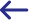# Return on Investment (ROI)

## What is Return on Investment?

Return on investment (ROI) is a performance measure used to evaluate the profitability of an investment or project. It is calculated by dividing the net profit of the investment by the total cost of the investment. The result is expressed as a percentage or a ratio.

## Challenges of Measuring ROI

Measuring ROI can be a difficult and time consuming task. There are a number of factors that must be taken into account, such as the initial cost of the investment, the time frame of the investment, and the amount of revenue generated. Additionally, there are several methods for calculating ROI, which can further complicate the task.

• Time Frame: Measuring ROI over a long period of time can be difficult. Over the course of several years, there could be changes in the market that could affect the profitability of the investment. This means that the ROI calculation must be adjusted accordingly.

• Initial Cost: The initial cost of the investment must be taken into account. The cost of the investment can fluctuate over time, which can make it difficult to calculate an accurate ROI.

• Revenue: The amount of revenue generated from the investment must be taken into account. If the revenue generated is too low, then the ROI will be negative.

• Methods: There are several methods for calculating ROI, such as the net present value (NPV), internal rate of return (IRR), and payback period. Each method has its own advantages and disadvantages.

## Overcoming the Challenges of Measuring ROI

The challenges of measuring ROI can be overcome by taking a few simple steps.

• Track Performance: Track the performance of the investment over time to get an accurate picture of the profitability. This will help to identify any changes in the market that could affect the ROI.

• Calculate Regularly: Calculate the ROI regularly to ensure that the results are accurate. This will help to identify any changes in the cost or revenue of the investment that could affect the ROI.

• Adjust for Inflation: Adjust for inflation when calculating the ROI. This will ensure that the results are not skewed by changes in the market.

• Use Multiple Methods: Use multiple methods for calculating ROI. This will allow you to compare the results and get a more accurate picture of the profitability of the invest

## The Benefits of Measuring Return on Investment:‍

• Compare Investments: Measuring ROI can help marketers and financial managers compare different investments. This can help them identify the most profitable investments and focus their efforts on those ventures.

• Make Better Decisions: Measuring ROI can help marketers and financial managers make better decisions when it comes to investments. It can help them identify investments that are likely to generate the highest profits and avoid those that are likely to lose money.

• Allocate Resources: Measuring ROI can help marketers and financial managers allocate resources more efficiently. It can help them identify which investments are most profitable and allocate resources accordingly.

• Maximize Profits: Measuring ROI can help marketers and financial managers maximize profits. By identifying the most profitable investments, they can focus their efforts on those ventures and maximize profits.

## How to Measure ROI

Measuring ROI is a relatively simple task. Here are the steps to calculate ROI:

• Determine Initial Cost: Determine the initial cost of the investment. This is the total amount of money invested in the venture.

• Calculate Net Profit: Calculate the net profit of the investment. This is the total amount of money made from the venture minus the initial cost.

• Divide Net Profit by Initial Cost: Divide the net profit by the initial cost of the investment. This will give you the ROI.

• Convert to Percentage: Convert the ROI to a percentage for easier comparison.

GO BACK